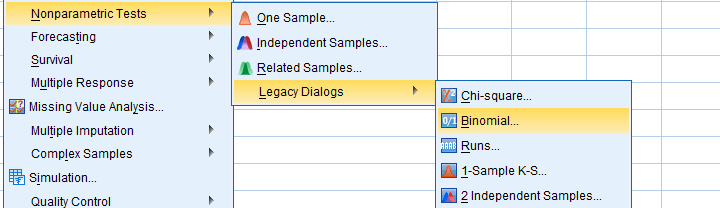SPSS TUTORIALS BASICS ANOVA REGRESSION FACTOR CORRELATION

# Overview SPSS Nonparametric Tests## Nonparametric Tests - One Sample

### SPSS Z-Test for a Single Proportion

Z-tests were introduced to SPSS version 27 in 2020.

This tutorial quickly walks you through z-tests for single proportions:

• which options should you select?
• how to interpret the output?
• which assumptions should you meet -and how to test these?
• and way more...

### Binomial Test – Simple Tutorial

A binomial test examines if a population percentage is equal to x.

Example: is 45% of all Amsterdam citizens currently single? Or is it a different percentage?

This simple tutorial quickly walks you through the basics.

### SPSS Binomial Test Tutorial

This tutorial walks you through running and interpreting a binomial test in SPSS.

### SPSS Sign Test for One Median – Simple Example

SPSS sign test for one median the right way. Recode your outcome variable into values higher and lower than the hypothesized median and test if they're distribted 50/50 with a binomial test.

## Nonparametric Tests - 2 Independent Samples

### SPSS Z-Test for Independent Proportions Tutorial

Z-tests were introduced to SPSS version 27 in 2020.

This tutorial quickly walks you through z-tests for 2 independent proportions:

• which options should you select?
• how to interpret the output?
• which assumptions should you meet -and how to test these?
• and way more...

### SPSS Mann-Whitney Test – Simple Example

The Mann-Whitney test is an alternative for the independent samples t test when the assumptions required by the latter aren't met by the data. The most common scenario is testing a non normally distributed outcome variable in a small sample (say, n < 25).

### SPSS Median Test for 2 Independent Medians

SPSS median test evaluates if two groups of respondents have equal population medians on some variable. This easy tutorial quickly walks you through.

## Nonparametric Tests - 3(+) Independent Samples

### How to Run a Kruskal-Wallis Test in SPSS?

The Kruskal-Wallis test is a nonparametric alternative for a one-way ANOVA.

This tutorial shows when to use it and how to run it in SPSS.

### SPSS Wilcoxon Signed-Ranks Test – Simple Example

SPSS Wilcoxon Signed-Ranks test is used for comparing two metric variables measured on one group of cases. It's the nonparametric alternative for a paired-samples t-test when its assumptions aren't met.

### SPSS McNemar Test

SPSS McNemar test is a procedure for testing whether the proportions of two dichotomous variables are equal. The two variables have been measured on the same cases.

### SPSS Sign Test for Two Medians – Simple Example

SPSS sign test for two related medians tests if two variables measured in one group of people have equal population medians.

### SPSS Friedman Test Tutorial

SPSS Friedman test compares the means of 3 or more variables measured on the same respondents. Like so, it is a nonparametric alternative for a repeated-measures ANOVA that's used when the latter’s assumptions aren't met.

### SPSS Cochran Q Test

SPSS Cochran's Q test is a procedure for testing whether the proportions of 3 or more dichotomous variables are equal. These outcome variables have been measured on the same people or other statistical units.

# Tell us what you think!

*Required field. Your comment will show up after approval from a moderator.

# THIS TUTORIAL HAS 7 COMMENTS:

•### By Monalisa on September 21st, 2018

Great help!

•### By Loo on September 24th, 2018

Hi Ruben, Post hoc test is available for chi square test of independence by using standard residual method, for example for a 3 by 3 chi square table. But, is it possible to do it for a 2 by 2 table?

•### By Ruben Geert van den Berg on September 24th, 2018

Hi Loo!

Post hoc tests examine which proportions differ if the main chi-square independence test indicates that 2 variables are associated.

For a 2 by 2 table, however, you're comparing only 2 proportions. So there's no question which proportions differ -there's only 2 of them.

On top of that, a z-test for 2 independent proportions is preferred over the chi-square test here. The significance levels are identical but the z-test provides a confidence interval of the difference too -which is very informative. For some stupid reason, it's missing from SPSS but we built a simple tool for it.

You can also inspect the (Pearson) correlation between the 2 variables. For some dumb reason it's called a phi coefficient for 2 dichotomous variables but it's technically identical to a Pearson correlation.

Last, Bonferroni corrected pairwise z-tests for larger (2 by 3, 3 by 3...) tables are often preferred over the adjusted standardized residuals. You can request the latter by adding BPROP to the CELLS subcommand as in

CROSSTABS education BY marital_status
/CELLS COUNT COLUMN BPROP
/STATISTICS CHISQ PHI.

Note that these tests only compare column (not row!) proportions.

Hope that helps!

SPSS tutorials

•### By Mohamed on November 10th, 2018

Hi there
What apout IRT in spss ver 15
Tell me
Thank you

•### By Ruben Geert van den Berg on November 10th, 2018

Hi Mohamed!

What do you mean by "IRT"? Item Response Theory? Please clarify.

Thx!

SPSS tutorials